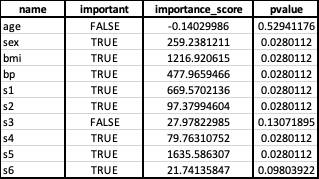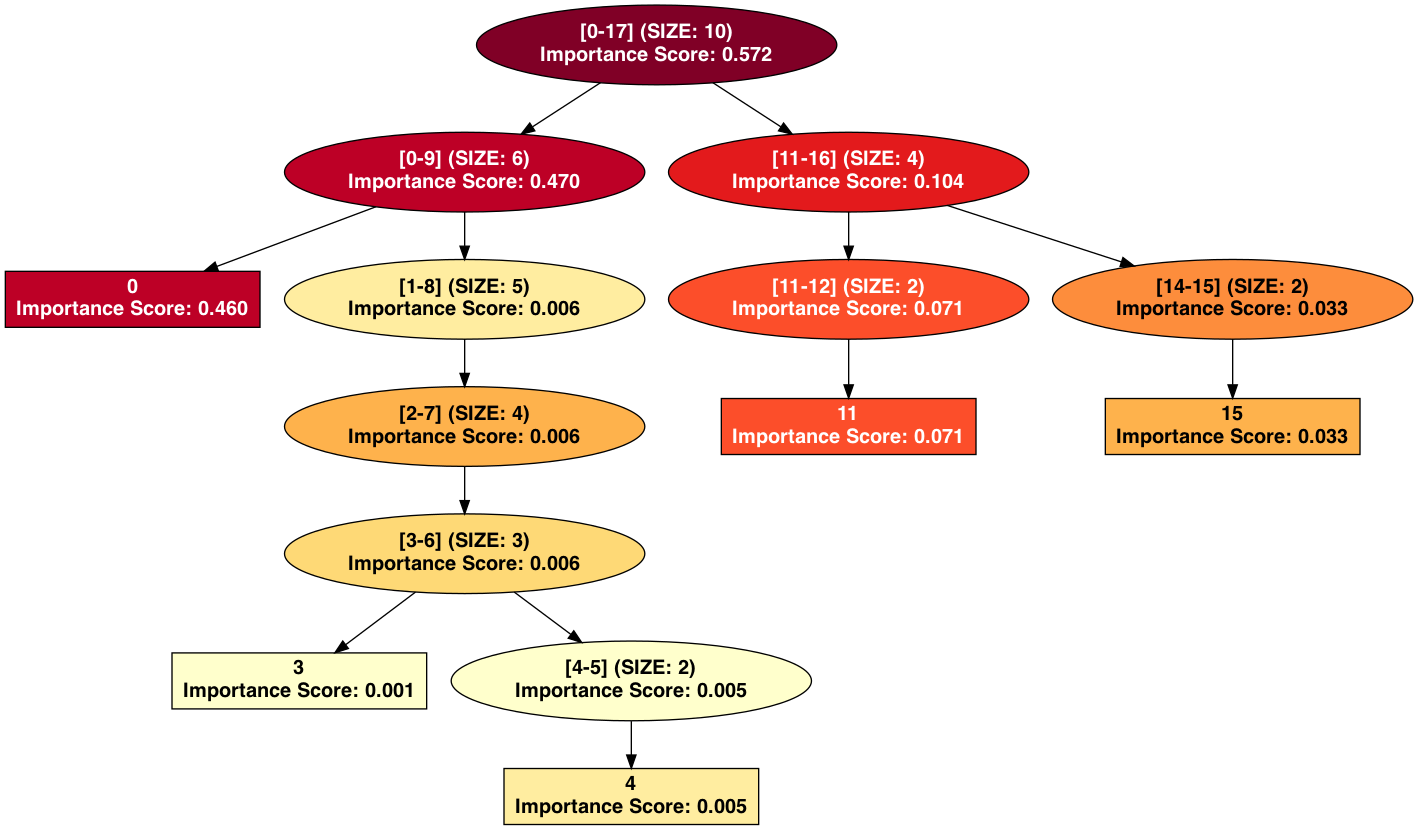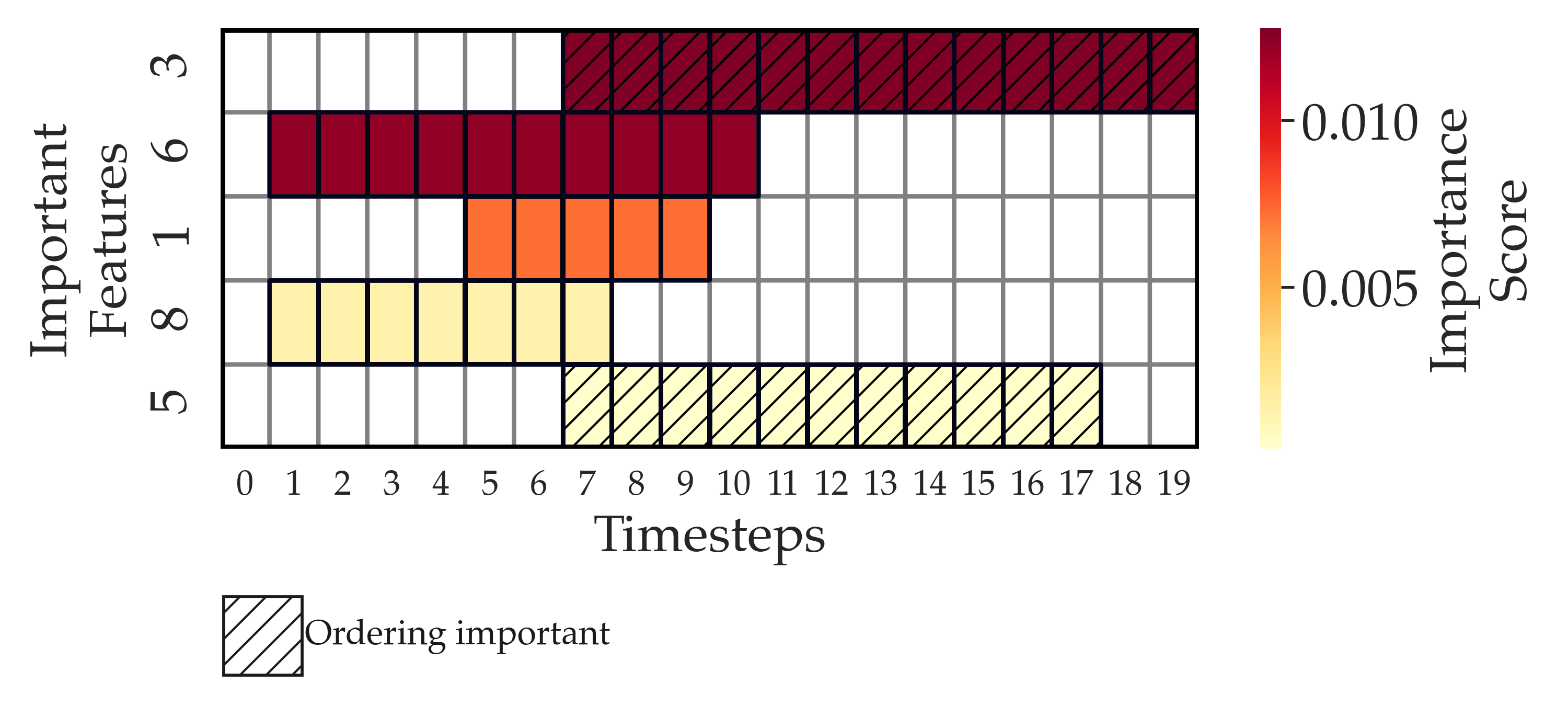Feature Importance Analysis of Models

## anamod### Overview

anamod is a python library that implements model-agnostic algorithms for the feature importance analysis of trained black-box models. It is designed to serve the larger goal of interpretable machine learning by using different abstractions over features to interpret models. At a high level, anamod implements the following algorithms:

• Given a learned model and a hierarchy over features, (i) it tests feature groups, in addition to base features, and tries to determine the level of resolution at which important features can be determined, (ii) uses hypothesis testing to rigorously assess the effect of each feature on the model’s loss, (iii) employs a hierarchical approach to control the false discovery rate when testing feature groups and individual base features for importance, and (iv) uses hypothesis testing to identify important interactions among features and feature groups. More details may be found in the following paper:

Lee, Kyubin, Akshay Sood, and Mark Craven. 2019. “Understanding Learned Models by
Identifying Important Features at the Right Resolution.”
In Proceedings of the AAAI Conference on Artificial Intelligence, 33:4155–63.
https://doi.org/10.1609/aaai.v33i01.33014155.
• Given a learned temporal or sequence model, it identifies its important features, windows as well as its dependence on temporal ordering. More details may be found in the following paper:

Sood, Akshay, and Mark Craven. “Feature Importance Explanations for Temporal
Black-Box Models.” ArXiv:2102.11934 [Cs, Stat], February 23, 2021.
http://arxiv.org/abs/2102.11934.

anamod supersedes the library mihifepe, based on the first paper (https://github.com/Craven-Biostat-Lab/mihifepe). mihifepe is maintained for legacy reasons but will not receive further updates.

anamod uses the library synmod to generate synthetic data, including time-series data, to test and validate the algorithms (https://github.com/cloudbopper/synmod).

### Usage

See detailed API documentation here. Here are some examples of how the package may be used:

Analyzing a scikit-learn binary classification model:

# Train a model
from sklearn.linear_model import LogisticRegression
from sklearn import datasets
model = LogisticRegression()
X, y, feature_names = (dataset.data, dataset.target, dataset.feature_names)
model.fit(X, y)

# Analyze the model
import anamod
output_dir = "example_sklearn_classifier"
model.predict = lambda X: model.predict_proba(X)[:, 1]  # To return a vector of probabilities when model.predict is called
analyzer = anamod.ModelAnalyzer(model, X, y, feature_names=feature_names, output_dir=output_dir)
features = analyzer.analyze()

# Show list of important features sorted in decreasing order of importance score, along with importance score and model coefficient
from pprint import pprint
important_features = sorted([feature for feature in features if feature.important], key=lambda feature: feature.importance_score, reverse=True)
pprint([(feature.name, feature.importance_score, model.coef_[feature.idx]) for feature in important_features])

Analyzing a scikit-learn regression model:

# Train a model
from sklearn.linear_model import Ridge
from sklearn import datasets
model = Ridge(alpha=1e-2)
X, y, feature_names = (dataset.data, dataset.target, dataset.feature_names)
model.fit(X, y)

# Analyze the model
import anamod
output_dir = "example_sklearn_regressor"
analyzer = anamod.ModelAnalyzer(model, X, y, feature_names=feature_names, output_dir=output_dir)
features = analyzer.analyze()

# Show list of important features sorted in decreasing order of importance score, along with importance score and model coefficient
from pprint import pprint
important_features = sorted([feature for feature in features if feature.important], key=lambda feature: feature.importance_score, reverse=True)
pprint([(feature.name, feature.importance_score, model.coef_[feature.idx]) for feature in important_features])

The outputs can be visualized in other ways as well. To show a table indicating feature importance:

import subprocess
subprocess.run(["open", f"{output_dir}/feature_importance.csv"], check=True)To visualize the feature importance hierarchy (since no hierarchy is provided in this case, a flat hierarchy is automatically created):

subprocess.run(["open", f"{output_dir}/feature_importance_hierarchy.png"], check=True)Analyzing a synthentic model with a hierarchy generated using hierarchical clustering:

# Generate synthetic data and model
import synmod
output_dir = "example_synthetic_non_temporal"
num_features = 10
synthesized_features, X, model = synmod.synthesize(output_dir=output_dir, num_instances=100, seed=100,
num_features=num_features, fraction_relevant_features=0.5,
synthesis_type="static", model_type="regressor")
y = model.predict(X, labels=True)

# Generate hierarchy using hierarchical clustering
from types import SimpleNamespace
from anamod.simulation import simulation
args = SimpleNamespace(hierarchy_type="cluster_from_data", contiguous_node_names=True, num_features=num_features)
feature_hierarchy, _ = simulation.gen_hierarchy(args, X)

# Analyze the model
from anamod import ModelAnalyzer
analyzer = ModelAnalyzer(model, X, y, feature_hierarchy=feature_hierarchy, output_dir=output_dir)
features = analyzer.analyze()

# Visualize feature importance hierarchy
import subprocess
subprocess.run(["open", f"{output_dir}/feature_importance_hierarchy.png"], check=True)Analyzing a synthetic temporal model:

# Generate synthetic data and model
import synmod
output_dir = "example_synthetic_temporal"
num_features = 10
synthesized_features, X, model = synmod.synthesize(output_dir=output_dir, num_instances=100, seed=100,
num_features=num_features, fraction_relevant_features=0.5,
synthesis_type="temporal", sequence_length=20, model_type="regressor")
y = model.predict(X, labels=True)

# Analyze the model
from anamod import TemporalModelAnalyzer
analyzer = TemporalModelAnalyzer(model, X, y, output_dir=output_dir)
features = analyzer.analyze()

# Visualize feature importance for temporal windows
import subprocess
subprocess.run(["open", f"{output_dir}/feature_importance_windows.png"], check=True)The package supports parallelization using HTCondor, which can significantly improve running time for large models. If HTCondor is available on your system, you can enable it by providing the “condor” keyword argument. The python package htcondor must be installed (see Installation). Additional condor options may be viewed in the API documentation:

analyzer = anamod.ModelAnalyzer(model, X, y, condor=True)

### Installation

The recommended installation method is via virtual environments and pip. In addition, you also need graphviz installed on your system to visualize feature importance hierarchies.

To install the latest stable release:

pip install anamod

Or to install the latest development version from GitHub:

pip install git+https://github.com/cloudbopper/anamod.git@master#egg=anamod

If HTCondor is available on your platform, install the htcondor PyPi package using pip. To enable it, see Usage:

pip install htcondor

### Development

Collaborations and contributions are welcome. If you are interested in helping with development, please take a look at https://anamod.readthedocs.io/en/latest/contributing.html.

anamod is free, open source software, released under the MIT license. See LICENSE for details.

Akshay Sood

## Project details

This version0.1.40.1.30.1.20.1.1

Uploaded source
Uploaded py2 py3